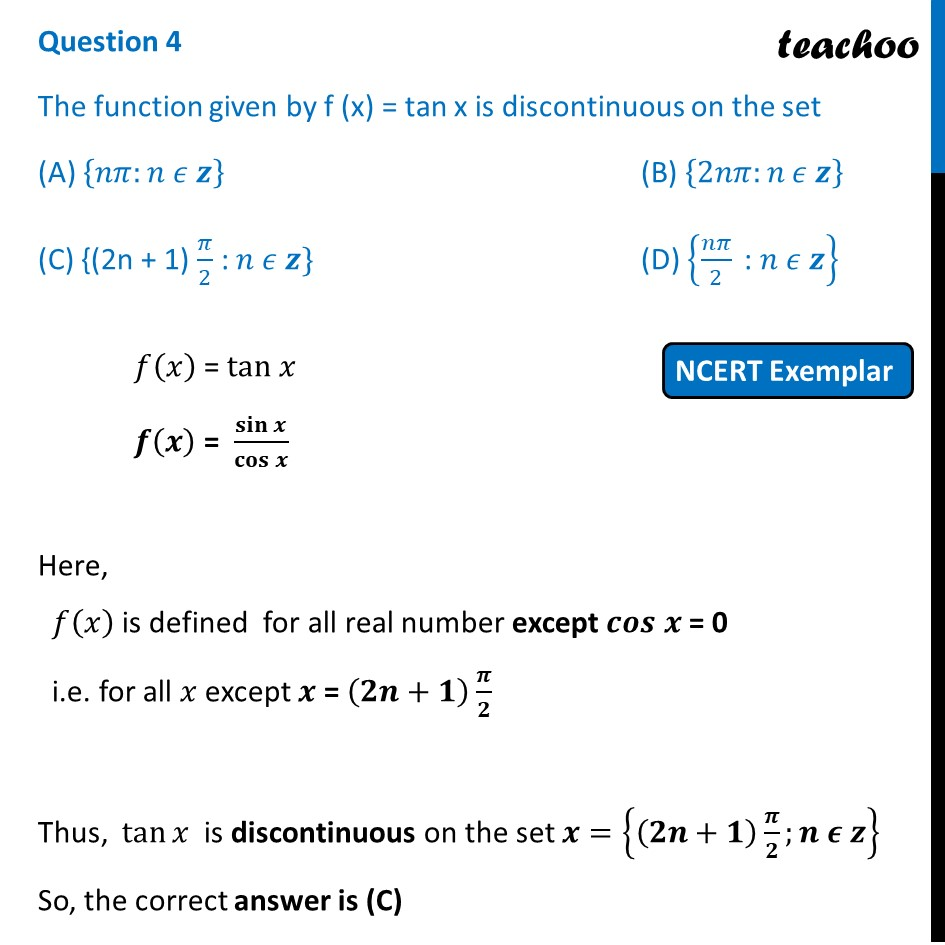NCERT Exemplar - MCQs

Chapter 5 Class 12 Continuity and Differentiability
Serial order wise

## (D) {nπ/2 " : " n ϵ z}

This question is similar to Example 18 - Chapter 5 Class 12  Continuity and DifferentiabilityLearn in your speed, with individual attention - Teachoo Maths 1-on-1 Class

### Transcript

Question 4 The function given by f (x) = tan x is discontinuous on the set (A) {𝑛𝜋:𝑛 𝜖 𝒛} (B) {2𝑛𝜋:𝑛 𝜖 𝒛} (C) {(2n + 1) 𝜋/2 : 𝑛 𝜖 𝒛} (D) {𝑛𝜋/2 " : " 𝑛 𝜖 𝒛} 𝑓(𝑥) = tan 𝑥 𝒇(𝒙) = 𝐬𝐢𝐧⁡𝒙/𝐜𝐨𝐬⁡𝒙 Here, 𝑓(𝑥) is defined for all real number except 𝒄𝒐𝒔 𝒙 = 0 i.e. for all 𝑥 except 𝒙 = (𝟐𝒏+𝟏) 𝝅/𝟐 Thus, tan⁡𝑥 is discontinuous on the set 𝒙={(𝟐𝒏+𝟏) 𝝅/𝟐;𝒏 𝝐 𝒛} So, the correct answer is (C)